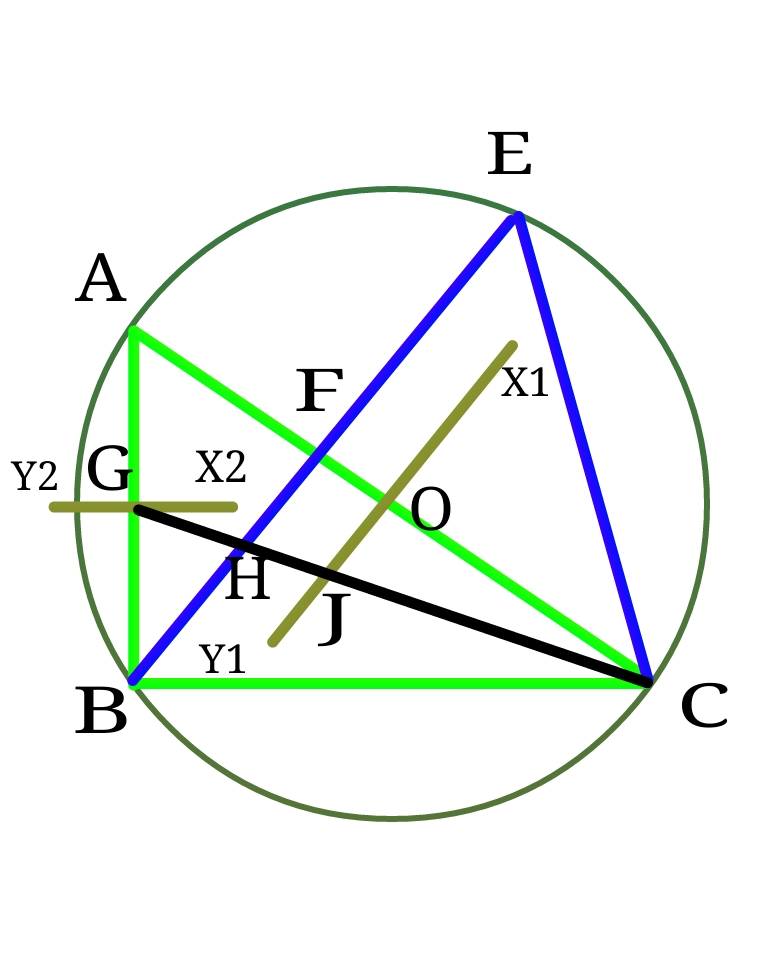# Construction Is A Piece Of Cake! Right? (Part-3)Mohit, influenced by Akshat and Anshuman, took a right $\bigtriangleup ABC$ (right angled at $B$), where $AB=5.5 \mathrm{ cm}$ and $BC=8 \mathrm{ cm}$, and started doing aimless constructions, the steps of which are given below:

($1$) He drew perpendicular bisector $X_1Y_1$ of $AC$ which intersects $AC$ at a point $O,$ and another perpendicular bisector $X_2Y_2$ of $AB$ which intersects $AB$ at a point $G$.

($2$) Then he constructed a circle taking center $O$ and radius of the circle as $OA$ . Then he constructed an $\angle ACE$ which is equal to $\angle ACB$ such that $E$ lies on the circle.

($3$) He then joined $BE$ which meets $AC$ at $F$.

($4$) Then he joined $CG$ which intersects $EB$ at $H$ and $X_1Y_1$ at $J$.

Mohit then wondered what $\frac{BH}{OJ}$ could possibly be equal to.

×